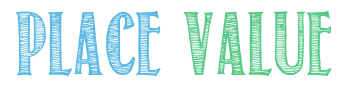## Get in touchWelcome to

Sawley Junior School

Aspire…Achieve…Thrive

## Get in touch

• Deputy Headteacher: Mrs L Taylor

# Maths

Miss Greygoose's Maths page

(If you ever lose of forget your password just ask me and I will give it to you!)Our next Maths topic is Fractions, Decimals and Percentages. We will be  comparing, converting and ordering fractions, decimals and percentages. The children will be building on their current equivalent fraction knowledge and identifying, naming and writing equivalent fractions of any given fraction. They will be converting between improper and mixed number fractions and using this to assist multiplying fractions by whole numbers.  Furthermore, they will be rounding decimals with two decimal places to the nearest one decimal place and whole number.Our next Maths topic is multiplication and division. We will be identifying multiples, factors and prime numbers, using the vocabulary prime factors and composite numbers. We will also be recognising square and cube numbers and solving multiplication and division problems. We will then be learning how to multiply and divide whole numbers and decimals by 10, 100 and 1000. After that, we will learn how to divide 4-digit numbers by 1-digit numbers and how to multiply 4-digit numbers by 1 and 2 digit numbers using the written method.Our next Maths topic is Statistics. We will be completing, reading and interpreting information in tables and time tables. We will also solve comparison, sum and difference problems using information presented in line graphs and charts.Our next Maths topic is Addition and Subtraction. We will be adding and subtracting numbers with more than 4-digits. We will be using rounding to check answers to calculations. As well as using column methods, we will be adding and subtracting mentally using increasingly large numbers. We will be solving multi-step word problems using appropriate operations and methods.

##Our first topic in maths will be all about Place Value. We will be reading, writing and comparing numbers up to 1,000,000 and learning what each digit represents, learning how to read and use Roman numerals, using negative numbers in context and practicing solving number problems.

## Ofsted

Trigger

We've had 1 0 5 7 3 1 visitors

Merits

• Barn Owls 32
• Eagle Owls 81
• Snowy Owls 75
• Tawny Owls 64

## Awards

Awards we have acheived so far.

Top